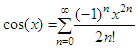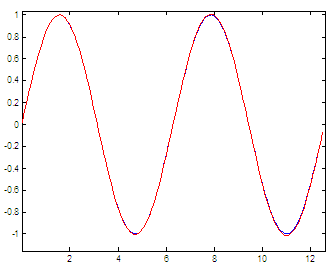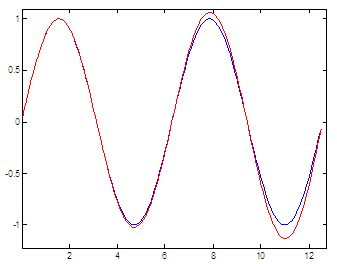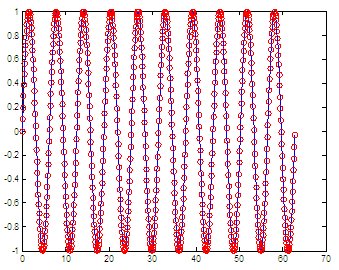# Sine series - working without the sine (or cosine) function

### Four ways to code a sine/cosine series in Matlab

 The sine function (usually expressed in programming code as sin(th), where th is an angle in radians) is one of the basic functions in trigonometry. In this article, we’re going to explore a number of ways to calculate the sine series without actually using the sine (or cosine) function. We’re going to play with the concepts of sine series, iterations, vectorizing programs... among others.

Sometimes we just can’t use a trigonometric function and we must use an alternative method to generate a table of values, for example.

The formulas that we are going to review and code are based on a few of the ideas expressed at mathworld.wolfram.com/Sine.html and mathworld.wolfram.com/Cosine.html.

### Experiment 1. Let’s code the cosine series to avoid using the cos(x) function

In this case the formula under study is the cosine series, defined asWe obviously can’t use an infinite number of terms, but we can see how accurate is our approach with a few terms. Our proposed function is

function y = cos1(x)
n = 0 : 100;
y = sum((-1).^n .* x.^(2*n) ./ factorial(2*n));

We’re not using for-loops (that’s vectorization), but we’re using some built-in functions such as sum and factorial.

We’re assumming that x is a scalar number, and our calculation includes only 100 terms. We’re just implementing the formula above... We must keep in mind that this is an approximation only.

If we test the code by comparing what the actual ‘cos’ function calculates against our result, then

x = 10;
cos(x)
y = cos1(x)

x = 40;
cos(x)
y = cos1(x)

We get from Matlab...

ans = -0.8391
y =   -0.8391

ans = -0.6669
y =   NaN

We can see that our result is good enough for the first case, but it’s not good for the second case. We must take into account that the built-in factorial function uses an algorithm with double precision numbers, and since double precision numbers only have about 15 digits, the answer is only accurate for N <= 21. We must be very aware of the limitations of our resources...

### Experiment 2. We’ll implement the sine series accepting a whole vector now

We’re going to produce a whole response now, not only a scalar. This function will receive a vector and will output another one. The sine series formula that we’re going to implement isOur proposed code in this case is the following

function y = sin2(x)
n = 1 : 100;
c = 2*n-1;
s = (-1).^(n-1);
f = factorial(c);

for i = 1 : length(x)
y(i) = sum(s .* x(i).^c ./ f);

end

We are just coding the formula... We calculate the three parts of the vectors: the alternating sign (s), the part that contains xi (within the loop), and the factorial part (f). Finally we multiply, divide and sum to produce every response corresponding to each element in the input vector. We have to iterate along all of the elements of the input vector to obtain all of the elements of the output vector. Again, we’re using n = 100, which seems to be a good enough number for our experiments...

We can fastly test the code, like this

x = 0 : .1 : 10*pi;
plot(x,sin(x),
'b', x,sin2(x),'ro')

We use horizontal values from 0 up to 10π, and we’re comparing the regular sine function (blue-solid line) against our experiment (sine series in red circles). The result is as followsThe approximation seems to work fine. Of course, we know that we need only values (responses) from 0 to
π/2 and that we can modify our algorithm to repeat those responses or change the sign as necessary, but we want also to simplify the code.

### Experiment 3. We’ll use a product instead of a sum to generate the sine wave

We’re going to implement another formula, shown hereIf we use n = 1000, we can implement an approach of the series in this way

function y = sin3(x)
n = 1 : 1000;

for i = 1 : length(x)
y(i) = x(i).*prod(1 - x(i)^2 ./ (n*pi).^2);

end

and a fast test is achieved in this way

x = 0 : .1 : 4*pi;
plot(x,sin(x),
'b', x,sin3(x),'r')

The result is shown in the following figureIf we used n = 100 only, we’d getThe blue line is the ordinary sine function; the red line is our approximation. We can see that the higher the x-value (angle) the higher number of n elements we have to have in order to obtain a good approximation. It’s all about accuracy in our response. It can deteriorate very easily...

### Experiment 4. We’ll use exponential functions to generate the sine wave

The definition of the sine function can be extended to complex arguments z, using the definition Another available formula isThis formula is very easy to evaluate if we have access to exponential functions and complex (imaginary) numbers. e is the base of the natural logarithm and i is the imaginary number.

The suggested code is very straightforward

function y = sin4(z)
y = (exp(i*z) - exp(-i*z))/(2i);

The equation is exact, it’s not an approximation. To test it we create a simple script like this

x = 0 : .1 : 20*pi;
plot(x,sin(x),
'b', x,sin4(x),'ro')

and our Matlab result isWe show 10 full cycles to show the accuracy of this method. The blue-solid line is the sine itself, and the red circles represent the implemented formula.

From 'Sine Series' to Matlab Examples home

From 'Sine Series' to 'Calculus Problems'